## 7.3 Furling, Unfurling& a Fractal Root

### A. A Reductionist Approach: Furling & Unfurling

#### Introduction

We are still interested in the reduction of our Directionals to the functions of the Raw data alone. At this point we've written an equation for the Decaying Average, the initial Derivative, in terms of the raw Data, see function F above, but the rest of our Directionals are written in terms of the Change Series and other Directionals. Although our cavemen can easily calculate these contextual Directionals, we as scientists want to reduce our Directionals to first principles, the Raw Data. Presumably reducing our Directionals to first principles will simplify our understanding. We will no longer need to calculate intermediate values. We will be able to go directly from the Raw Data, right to the Directional, with no need for intermediate steps. At present our Directionals are written in contextual terms. We want to sink below the context into content. We want to reduce our equations to their simplest terms, the raw Data. This is a reductionist approach. We proved the existence of the G & H functions above. The G function writes the First Directional in terms of Raw Data. The H function writes the General Directional in terms of the Raw Data. In the reductionist approach we hope to be also pursuing simplicity. So far the reductionist approach has only led to increasing complexity. Although aware of the possibility of increasing complexity, we proceed on in our quest to find the G and H functions for the First and General Directional.

#### Notational Simplifications

Let us introduce a notational simplification for the general Directional.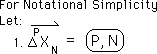#### Definitional Equation for the General Directional

Below is the definitional equation for the general Directional. D is the Decay Factor, a positive integer. K, another constant, is the diminishment factor. It is always less than 1 and greater than or equal to 0. So K diminishes whatever it comes in contact with as a product.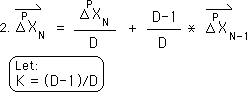Below is the rewriting of the general formula in the new less cumbersome notation.Below is the extension of the F function with nested f functions suggested in equation #13 on page 3. The expressions unfurl.#### The Unfurling Function

Let us furl them back up, ready to be unfurled by the Unfurling Function. Below is the definitional formula for the Unfurling Function.Our General Directional is furled up in the expression below by the Unfurling Function. This is the General Furled Equation for the Directionals.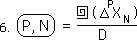#### Furling the Decaying Average

Below is the application of the General Furled Equation to the situation when P = 0. When P = 0, we are talking about the zeroth Directional, the Initial Derivative, in other words, the Decaying Average.Remember from the Derivative Notebook that the zeroth level of change is the Data itself. This is technically, not a derivative, but the Source of all the Derivatives. It is derived from a measurement of Reality. We will call it the Source Derivative.We see that the Decaying Average is simply the Furled Data divided by D, the Decay Factor.#### The Fractal Root Pattern, linking the Data & the Average

Let us introduce one more notational simplification that will prove useful to our derivation.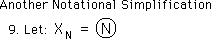Below is its application to our Furled equation for the Decaying Average. This equation will prove to be very important because it is at the fractal root of our unfurling fern. It is the Fractal Root Pattern.#### The First Directional, Furled

Applying the General Furled Equation to the First Directional, we get the following expression.Remembering the definition for the first level of change, we substitute this expression into equation 11 and it becomes equation 12.In equation 13, we convert to our circular notation. We come to a block because we are not sure if the Distributive law applies to our Unfurling Function.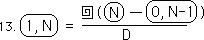### B. The Distributive Law for the Unfurling Function

The next section proves the Distributive law for the Unfurling Function. Because the Unfurling Function only unfurls the N part of the General Directional and has nothing to do with the P part, we will let the circled N represent the Nth member of the General Directional with a power of P. For the same reasons we will let the circled M represent the Mth member of the General Directional with a power of Q. Normally the circled N and M refer only to Raw Data. In this proof only they represent the more general directional.Although the situation is reversible, in this case, we will let N ≥ M.Let us Unfurl (N - M) according to the definition of unfurling, Equation #5 of the previous section.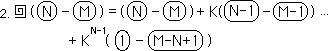We then separate out the N and M parts. They are not at all connected because there are no products between them.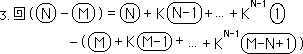Because N ≥ M, M-N≤ 0. Therefore X with a subscript of M-N will equal 0.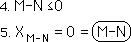Let A be an integer such that: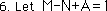Then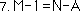Let B be an integer less than A.Then it follows that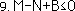Therefore a Data Point, X, with such a subscript will equal zero. There are no Data Points before the first.Therefore a Data Point, X, with a subscript of M-N+A will be the first Data Point, X1.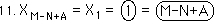For the N Stream we are already back to the first data point by design. For the M stream, the above expression is the first Data Point because all the prior terms equal zero. We also substituted the appropriate symbols in the exponent for the last K.From Equation 7 above we make another substitution for K's exponent.Both the N Stream and the M stream can be furled separately by the Unfurling Function.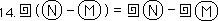This leaves us with our proof that the Unfurling Function obeys the Distributive Law across addition. Remember that the circled N and circled M represent the general Directionals not just the Data. Therefore the Distributive Law applies to Unfurling Function for all levels of Directionals.

### C. Back to the First Directional: Introducing Questions

#### A Double Unfurling?

Let us return to Equation 13 of the section before the last. We can now apply the distributive law to the Unfurling Function with the result below.Substituting the Fractal Root Pattern, Equation 5, for the Decaying Average, we get the following equation.Combining terms, we get a double unfurling function.This leads to the question, what does a double unfurling mean?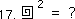Taking another tack we return to equation 14 and break it into two parts.

#### The Decaying Average to the 2nd Power?The first term is the Fractal Root Pattern, which is the Decaying Average. The second term is the Fractal Root Pattern applied to the Decaying Average. In the second term, the circle becomes the F function.We introduce another notational simplification, circle squared.Using the above notation our equation for the first Directional becomes the difference between the Decaying Average of the Nth Point and the Decaying Average to the second power of the prior point.The next question is what does it mean to take the Decaying Average to the second power?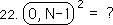We fold up our equation for the first Directional, into the Delta function.

#### The First Directional as a function of the Decaying Average and Delta?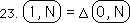This leads to a few more questions. What does it mean to fold up our expressions? And why do we want to fold them up?In the next few sections we will deal with the questions raised above: Double Unfurling, Folding Functions, and an Unraveling Function.